# Introduction to Deep Learning for Computer Vision

Christian Zuniga, PhD

Introduction

Neural networks have in the past decade achieved high, even super-human accuracy, at many computer vision tasks such as image classification, object detection, and image segmentation. Although neural networks and the backpropagation training technique have been available since the 1980’s, recent advances in hardware like GPUs, architectures, algorithms, and availability of large high quality labeled data have allowed neural networks to reign supreme on these tasks. For example, in the ImageNet data set, a program has to correctly classify an input image from 1000 categories. In 2012, a breakthrough was made when the classification error was lowered from 26% to 16% using a particular kind of neural network called a convolutional neural network or CNN . Improved network architectures since then have lowered the error to less than 5% as shown in Figure 1 .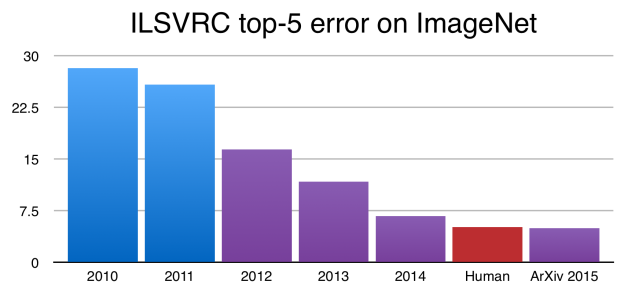Figure 1 Classification error on ImageNet data set over several years. 2012 shows a noticeable reduction in error.

Figure 2 shows a typical architecture of a CNN . It consists of two major blocks, an image feature generator, and an image classifier. The raw image is not in a suitable form to classify it directly so salient characteristics or features are first extracted. The feature generator convolves an image with multiple kernels and nonlinearly transforms this output to generate features. This process may be repeated several times to generate the final features. These are then down sampled and flattened into a vector before entering the classifier. This second component predicts the class of the image using the generated features. It consists of a traditional feedforward neural network that will be explained later in this article. Both the feature generator and image classifier have parameters that need to be optimized by a training process to correctly predict an image class.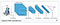Figure 2 Typical architecture of a Convolutional Neural Network.

FIn general any image classifier (not only neural networks) first learns how to classify an image using many pre-classified image examples, a process called supervised machine learning. In this process people first collect a training set of images and manually label each one. Then in the training stage, the classifier’s parameters are tuned or optimized so that it correctly categorizes an input image using the known labels.

For example, the MNIST data set consists of images of the digits from 0–9 giving 10 classes . It has 5000 different images for each digit for a total of 50,000 training images. Figure 3 shows a sample of the images. Each image in the training set has a 10-element target vector yi representing its class. This is called a one-hot encoding scheme. The class vector has a 1 at only one of the 10 positions, and the rest of the elements are 0. For instance, the digits 2 and 6 are represented by: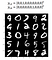Figure 3 Sample images from MNIST

The image classifier also outputs a 10-element vector yp giving a probability for each of the classes. The cross entropy loss function quantifies the difference between the target y and prediction yp. The loss L is 0 if the classifier’s prediction agrees with the target and is greater than 0 if it does not. It is based on maximizing the likelihood function, or p(y|parameters), using the Bernoulli distribution .

The prediction yp is a function of the classifiers parameters P or yp = f(P). Optimization finds the best parameters by minimizing the cross entropy through an iterative algorithm called gradient descent. The gradient of L with respect to the parameters gives the direction of increasing L. To decrease L, the opposite direction is followed as shown in Figure 4 for the 1-parameter case. In its simplest form gradient descent finds the parameters by the equation below. ADAM is a more advanced optimization method. The parameters have some reasonable initial values P. The hyperparameter alpha is called the learning rate and is optimized externally.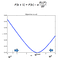Figure 4 1-dimensional example of gradient descent optimization.

The algorithm just described is for stochastic gradient descent where 1 image is used to calculate the gradients. It is usually better to divide the training data into mini batches of size N. The gradient would then be an average over the mini batch examples.

For CNNs, the parameters of the feature generator are also automatically optimized in the training. Prior to deep learning, features like edges and corners were manually generated using expert knowledge. Deep learning automates the feature generation process and has shown to result in better accuracy. Once a CNN is trained, its parameters are fixed, and it can then by applied to new images, not used during training to accurately predict their class.

CNN Training

Although there are modern frameworks such as TensorFlow  to handle the training, it is instructive to understand neural network training in greater detail and open the black box. Backpropagation is a dynamic programming algorithm used to rapidly train a neural network and was discovered in the 1980s . It is essentially based on the chain rule of calculus. To understand backpropagation, it is first necessary to understand the operations of a neural network.

Forward Propagation

Every neural network is made up of computing units called neurons. A single neuron is shown in Figure 5. It consists of a set of weights wf, a bias b, and two operations, a summation and a non-linear transformation. The weights and biases of all neurons make of the parameters that need to be optimized. The neuron non-linearly transforms an input vector x into an output value a. First the input vector elements are linearly combined with the weights and a bias is added to produce an intermediate output g. Then g is passed through a non-linear function f to produce the output a. The function f is a non-linear function called an activation function and is necessary to give the network rich learning abilities. For example, new frequencies can be produced.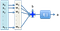Figure 5 Schematic of a single neuron.

There are several choices for activation functions including sigmoid, tanh, and ReLu. Traditionally the sigmoid was used but has been replaced by the ReLu function shown in Figure 6. It and its derivative are easy to compute and the derivative does not saturate as other functions.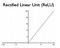Figure 6 Diagram of ReLu = max(0,g).

The operation of a neuron can be more compactly expressed as a standard matrix multiplication as shown below. The first operation is a multiplication between the row weight vector w and column input vector x. This is also the inner product between two vectors.

In a feed-forward neural network multiple neurons are arranged in a layer as shown in Figure 7. Each oval is a neuron and operates on the input vector x. There are no connections between the neurons. The weights are now contained in a matrix W. The ith row contains the weights for the ith neuron. The number of columns equals to the number of elements in x. The intermediate output becomes a vector g. The activation function operates on each element of g to make an output vector a.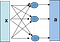Figure 7 Schematic of multiple neurons arranged in a single layer.

A neural network can have many layers as shown in Figure 8 and multiple layers is what makes it a deep neural network. Each layer k operates on the vector output of the previous layer as shown below. Each layer has Nk neurons. If there are K layers, there are K weight matrices, each of dimensions (Nk, Nk-1).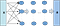Figure 8 Multi-layered neural network.

Using Python’s Numpy library , these operations can be conveniently expressed as:

The last layer gives the output and its form depends on the task being done. For classification a soft-max layer is frequently used and replaces the activation function f. Given C classes, the output of this layer gives the probabilities the input x belongs to each of the C classes.

The feature generator in a CNN also consists of neurons but have some constraints. As previously mentioned, an input image of size (Ni, Ni) is convolved with a kernel, typically of size (3,3) to make a feature (or feature map). The basic operation is shown below.

The convolution differs from the traditional definition of convolution in signal processing and consists in sliding the kernel w across the image without flipping. At each position a linear combination of the image values and weights of the kernels is made. Many excellent references further describe this operation in detail . For the purposes of this article, it is useful to represent the convolution using matrix multiplication. The image is turned into an (Ni^2, 1) vector by stacking the rows into columns. The kernel is turned into a weight matrix by placing the weights appropriately in the matrix. A (3,3) kernel will be used as an example. The 2D-convolution is then made with a matrix multiplication.

For example if the image was (5,5), it would be turned into a (25,1) vector and the weight matrix would be of size (25,25) of the form below. The weight matrix will be very sparse for small filter size and large images. Stride lengths greater than one and zero padding will change the position of the weights.

Instead of using one matrix W, it is better to use 9 shift matrices (for 3x3 filters). Each shift matrix has only one diagonal equal to 1 at the appropriate non-zero positions and the rest of the elements are 0. The derivatives will turn out to be very simple . The convolution matrix can then be expressed as the weighted sum of 9 shift matrices.

For example, if a convolution matrix was of size (4,5), a shift matrix could be.

An input vector i=[i0, i1, i2, i3, i4]^T would be shifted and truncated to

g=[i1, i2, i3, i4]^T.

The actual shifting operation could then be implemented by slicing an array instead of a matrix multiplication. If s indicates an upward shift (for column vectors) and 3 shifts were needed, then they could be made by:

If all shifts are stored as columns in a matrix Is and the 9 weights broadcasted to a matrix Ws, then the convolution result can be calculated as an element wise multiplication g=np.multiply(Ws,Is).

Another difference in the convolution used for CNNs is that the neurons are always fully connected across depth. A color image may have at least 3 channels (Red, Green, and Blue) in which case the filter is applied to each channel and the results added up. The weight filters are now 3-dimensional of size (3,3,3). In general, the filter size would be (f, f, Nc) where Nc is the number of channels. However it is easier to think of matrices and vectors. Multiple filters may also be applied to the image giving multiple features. Each feature may be passed through a non-linear activation function f as before. Bias is neglected for simplicity.

A CNN has another operation called pooling that down samples the features. Frequently max pooling is used where the maximum of a1,f within a pooling window is chosen. This operation can preserve translation invariance making it useful for feature detection. Once the indices of the maximum values within each window are identified, the pooling operation can also be thought of as a matrix multiplication. The backpropagation will then simply use the transpose of the matrix. The rectangular matrix D has values of 0 or 1 with a 1 selecting the maximum values.

There may be more convolutional layers of this form (convolution + non linear activation + pooling) before reaching the fully connected classifier. The output vector at layer k, filter i is given below. Layer k-1 has Nk-1 features. Figure 9 shows the computational flow where each rectangle represents a feature map.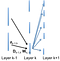Figure 9 Example of computational flow in 3 CNN layers.

The last output layer of the feature generator is flattened to form an input vector x for the classifier.

Backpropagation

As previously mentioned, the weights and biases are found by gradient descent as shown below for the weights. There is a similar equation for the biases.

Backpropagation speeds up computation of the partial derivatives by repeatedly using the chain rule. The gradient is shown below in matrix form. 

The vector sk is an (Nk, 1) sensitivity vector of the loss function L with respect to the intermediate output gk. As the third equation shows, the sensitivity vector at layer k can be calculated using the sensitivity of the layer in front, layer k+1, saving computation time. Backpropagation starts from the output layer and flows backward through the network. With a softmax output and cross entropy loss, sK = yp - y.

The matrix F’ is a diagonal matrix containing derivatives of the activation function in the diagonals. For a ReLu function these would be either 0 or 1.

The backpropagation step is also easily implemented with Numpy as shown previously. The intermediate outputs need to be stored. When the feature generator is reached, the equations are modified slightly. There are fewer parameters since the weights are shared among neurons. The shift matrices introduced earlier give a very simple form for the derivatives. They involve shifting of the input vector like the forward propagation step. There are only 9 derivatives for a 3x3 filter.

Each feature receives multiple sensitivities from the layer in front as shown in Figure 10. The transpose of the pooling matrix transforms the vector back to the larger size.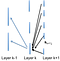Figure 10 Example of backpropagation flow through CNN layers.

Once all the sensitivities are calculated, the weights are updated. The process of forward propagation and backpropagation is then repeated for a specified number of iterations. A validation set of data could be used to stop the process early if the validation error does not improve or increases. With mini-batch gradient descent the gradients are calculated from the average of the mini batch. Each complete pass through all mini batches is called an epoch.

The trained CNN can then have the weights fixed and used on new images not in the training set. A CNN trained on MNIST may not be good enough for other types of images since the features it learned are more limited. It will most likely do well with digit recognition. CNNs trained on ImageNet, a set having a much richer set of images, have shown to be useful with other types of images. Only the last few classifier layers are trained and the feature generator is fixed ( a process called transfer learning).

References

 Murphy “Machine Learning” A Probabilistic Perspective, MIT Press 2012

 Strang “Linear Algebra and Learning from Data” Cambridge Press 2019

 Hagan, et. al. “Neural Network Design” 2nd Edition, 2014

Christian Zuniga has worked as a lecturer in San Jose State University in data mining. He worked in OPC modeling and is interested in machine learning.

## More from Christian Zuniga

Christian Zuniga has worked as a lecturer in San Jose State University in data mining. He worked in OPC modeling and is interested in machine learning.